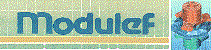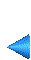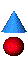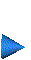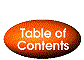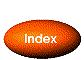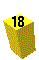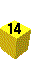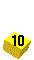## 1.4.3 Implementation

This program is based on keywords and requires a data file containing the corresponding list of keywords and associated values. There is an option to create the data file (or commands file), and another to read this file and activate the requests which are stored in it.

### Calling, libraries and files

The MODULEF library [Bernadou et al. 1988] is organized into several sets of programs or (sub-)libraries. Each of these contain the programs corresponding to one predetermined type of application. In this way, the general utility programs are in found library UTIL, those dedicated to the manipulation of data structures are found in UTSD, the mesh generators and modules treating two-dimensional meshes are found in NOP2, those treating three-dimensional meshes are in NOP3, the non- dedicated mesh application programs are found in NOPO, etc., the main programs are in PPAL, the utilities employed in the conversational (interactive) system are in CONV and CONW, etc.

There are two ways of calling module APNOPO:

1. via preprocessor APNOXX, or
2. directly.

In the first case, we must perform a link between libraries CONV, CONW, NOP2, NOPO, UTSD, UTIL and UTIF (general non-portable utilities) and program APNOXX .

In the second case, the libraries required are the same as above, except for CONV and CONW, which are now inutile.

A calling program, in FORTRAN 77, must be written in the following manner:

• COMMON M(LM)
Declaration of the super-array M, of LM words
• EXTERNAL FFRONT
Declaration of the "function"
• CALL INITI(M,LM,IMPRE,NNN)
Initialization for all MODULEF jobs
• CALL APNOPO(M,M,FFRONT)
Call the module
• STOP
• END

The only arguments of this module are the super-array, M, and the formal name of a possible projection function. The data is contained in a command file in the form of keywords and associated values.

The list of keywords, classed according to the level mentioned previously, is given below. For each value, the corresponding type expected is stipulated ((I): Integer, (R): Real, (A): Character, etc.).

### Keywords

COUR : Definition of the curves present in the domain via a function parser.

This data is used when the lines are constructed or the mesh is repartitioned.

• COUR  (A) : the keyword to activate
• IMPRE (I) : print parameter for execution
• Loop from J=1 to the number of descriptions:
• 'COURBNN(X,Y) = X*X + (Y-2)*(Y-2) -0.125;'
The equation of the curve is referenced by number NN.
The equation must be enclosed by ' and terminated by a ; character.
• FIN (A) : indicates the end of data for this keyword.

Remarks:

• If we utilize function FFRONT, this keyword is inutile.

• The curves may have an arbitrary number NN (from 1 to 99). Curve number NN is used once the lines (or edges) with reference NN are encountered (see keywords LIGN et RETR).

POIN : Definition of the characteristic points .

• POIN  (A) : the keyword to activate
• IMPRE (I) : print parameter for execution
• NPOINT (I) : number of points to describe
• Loop from J=1 to NPOINT, the number of characteristic points
• NOP (I) : the point number
• NOREF (I) : its reference
• X (R) : its abscissa
• Y (R) : its ordinate
• end of loop J

Remarks:

• The points may be entered in any order (due to NOP).
• If the points do not lend itself to a manual capturing (due to their number or position), but are easier to capture via a subroutine, we can use subroutine SPDPTS called by module APNOPO. This is done by:

• fixing the value of NPOINT to -NPOINT and omitting the loop,
• supplying subroutine SPDPTS as follows:

SUBROUTINE SPDPTS(NPOINT,MPPOI,MNRPC,XY)

where

NPOINT
is the number of points (input),
MPPOI(*)
is the integer array linking local point I to global point J=MPPOI(I),
MNRPC(*)
is the array of point references (indexed by I), and
XY(2,*)
is the real array of point coordinates (indexed by I), i.e.,
X = XY(1,.), Y = XY(2,.).

LIGN : Definition of the characteristic lines  from the characteristic points (input beforehand via keyword POIN).

Each line is constructed from characteristic points and is partitioned into segments in accordance with the prescriptions contained in its description.

• LIGN  (A) : the keyword to activate
• IMPRE (I) : print parameter for execution
• NDLM (I) : the number of lines to be described
• Loop from J=1 to NDLM, the number of characteristic lines
• NOLIG (I) : the line number
• NOELIG (I) : the number of points on the line (extremities included)
• NEXTR1 (I) : the number of the point at endpoint 1 of the line
• NEXTR2 (I) : the number of the point at endpoint 2 of the line
• NOREF (I) : the line reference
• NFFRON (I) : the description option relating to the line geometry (see below)
• RAISON (R) : the geometric progression ratio between the points to be generated on the line
• if NFFRON = -1, loop from K=1 to NOELIG -2
NOEL(K) (I) : the point numbers (extremities excluded) on the line

• if NFFRON = -3:
NOCE (I) : the number of the circle center (in the list of characteristic points)

• end of loop J

Remarks:

• The lines can be input in any order (due to NOLIG).
• NFFRON specifies the geometry of the line (i.e. the manner in which the intermediate points are created). There are 5 options:

1. NFFRON = 0 : The line is a straight line connecting points NEXTR1 and NEXTR2;
2. NFFRON > 0 : the line is described by function FFRONT for index NOREF or by the curve, COURBEJJ with JJ = NOREFL.
In this case, the line must be described in the trigonometrical direction.
3. NFFRON = -1 : the line is defined as the set of segments formed by the points NEXTR1NOEL(1), NOEL(1)NOEL(2), ...
..., NOEL(NOELIG-2)NEXTR2.
4. NFFRON = -2 : the line is a circle centered at the origin and passing through points NEXTR1 and NEXTR2 (note the direction).
5. NFFRON = -3 : the line is the circle centered at one of the characteristic points (where we give the number NOCE) passing through points NEXTR1 and NEXTR2 (note the direction).

• For NFFRON = -2 or -3, we define a circle.

Warning: This information is only used to construct the mesh of the lines and is discarded thereafter. Consequently, this does not prevent giving a non-zero reference to the line and entering the associated equation of the circle (keyword COUR) which, having done this could be used, for example, when repartitioning the mesh or adding nodes (keywords RETR or ADPO).

Module COLIGN, called by APNOPO, use the definition of the lines to construct their discretized form, i.e., the set of NOELIG - 1 segments of the corresponding straight lines.

The lines enable us to define the contours (discretized) of the domains to mesh. A contour has the form of an assembly of lines, and thus the assembly of their items.

QUAC : Call the mesh generator QUACOO which corresponds to an algebraic method for the case of a generalized quadrangle. The input data consists of the contour of the domain in terms of lines (keyword LIGN).

• QUAC  (A) : the keyword to activate
• IMPRE (I) : print parameter for execution
• LEVEL (I) : the level of the resulting D.S.
• NUDSD (I) : the sub-domain number to assign to the elements created
• NBRELI (I) : the number of lines constituting the contour of the domain
• NS1L (I) : the direction of the first line of the contour: if NS1L = 1 , the line runs from NEXTR1 towards NEXTR2, if NS1L = -1, the line runs in the opposite direction (i.e. from NEXTR2 to NEXTR1)
• Loop from J=1 to NBRELI, the number of lines constituting the contour
• NOLIG(J) (I) : the number of line J
• end of loop J
• IMAX (I) : the number of points on the first side of the quadrangle to be meshed (endpoints included)
• NQUAD (I): element splitting option:
• 1 : mesh consisting of quadrangles,
• 0 : mesh consisting of triangles (choice of the best angle to cut the quadrangles),
• -1 : same, with special treatment of the topological corners of the initial quadrangle,
• -2 (-3) : regular mesh (choice of the same diagonal for each splitting of quadrangles) with special treatment at the corners,
• -4 (-5) : regular mesh without special treatment at the corners.

Remarks:

• Two consecutive lines must have a common endpoint.
• The number of points on the contour must be even. In fact, the domain is considered as a quadrilateral and the number of points on two opposite sides must be the same. Consequently, the knowledge of IMAX is sufficient to determine the splitting completely.

• IMAX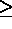2, same for JMAX (the equivalent of IMAX for the 2 other sides, calculated implicitly).

Figures 1.2 to 1.5 shows the effect of parameter NQUAD.

fig5bd.psfig:quac1Regular splittingfig5be.psfig:quac11Other choice

fig5bf.psfig:quac2Other splittingfig5bh.psfig:quac21Other choice

TRIC : Calls the mesh generator TRICOO which corresponds to an algebraic method  for the case of a generalized triangle. The input data consists of the contour of the domain in terms of the lines (keyword LIGN).

• TRIC  (A) : the keyword to activate
• IMPRE (I) : print parameter for execution
• LEVEL (I) : the level of the resulting D.S.
• NUDSD (I) : the sub-domain number to assign to the elements created
• NBRELI (I) : the number of lines constituting the contour of the domain
• NS1L (I) : the direction of the first contour line: if NS1L = 1 , the line runs from endpoint NEXTR1 towards endpoint NEXTR2, if NS1L = -1, the line runs in the opposite direction (i.e. from NEXTR2 to NEXTR1)
• Loop from J=1 to NBRELI, the number of contour lines
• NOLIG(J) (I) : the number of line J
• end of loop J
• IMAX (I) : the number of points on the first side of the triangle to mesh (endpoints included)

Remarks:

• Two consecutive lines must have a common endpoint.

• The number of points on the contour must be a multiple of 3. In fact, the domain corresponds to a triangle and the number of points on its topological sides must be equal. Consequently, the knowledge of IMAX suffices to determine the splitting completely.
• IMAX2.

TRIA : Calls the mesh generator TRIFRO which corresponds to a advancing-front method . The input data consists of the contour of the domain in terms of lines (keyword LIGN). Internal points can also be specified.
Furthermore, this mesh generator accepts contours with several components ; it allows to generate triangles or quadrangles meshes and/or adaptive meshes.

• TRIA  (A) : the keyword to activate
• IMPRE, NIVEAU, NUDSD, NBRELI, NPROPA as for keyword TRIH
• Loop from J=1 to NBRELI the number of contour lines
• NOLIG(J) (I) : the number of the line J of the contour.
• end of loop J
• NCOMP, NBRINT like the keyword TRIH
• IOPT (I) : front management option (i.e. selection of the departure segment)
• 0 : mesh consisting of triangles - slow option
• 1 : mesh consisting of triangles - fast option (this option is advised)
• 10 : mesh consisting of triangles - slow option - adaptive version
• 11 : mesh consisting of triangles - fast option - adaptive version
• 20 : mesh consisting of quadrangles - slow option
• 21 : mesh consisting of quadrangles - fast option
• 30 : mesh consisting of quadrangles - slow option - adaptive version
• 31 : mesh consisting of quadrangles - fast option - adaptive version
• if IOPT = 10, 11, 30 or 31
• NOMFIC (C) : name of the file that contains the initial mesh
• NIVEAU (I) : level of this mesh
• NOMFIC (C) : name of the file that contains the data structure B, this data structure B contains for each point of the initial mesh the size of the cells in its neighborhood
• NIVEAU (I) : level of this data structure

• Loop from K=1 to NCOMP the number of contour components
• NTACO(K) (I) : the number of lines of component K of the contour (see TRIH).
• end of loop K
• Loop from L=1 to NBRINT the number of fixed internal points (if > 0)
• NUMPIN(L) (I) : the internal point number L (see TRIH) ;
• DIAM(L) (R) : the desired diameter for the triangles created around point L.
• end of loop L

Remarks:

This keyword requires the same data than the following keyword (TRIH), except the IOPT parameter describe above.

TRIH : Calls the mesh generator TRIHER which corresponds to a Voronoi method . The input data consists of the contour of the domain in terms of lines (keyword LIGN). Internal points can also be specified.
Furthermore, this mesh generator accepts contours with several components. It allows to generate adaptive meshes. In its standard version (non adaptive) internal edges can also be specified.

• TRIH  (A) : the keyword to activate
• IMPRE (I) : print parameter for execution
• LEVEL (I) : the level of the resulting D.S.
• NUDSD (I) : the sub-domain number to assign to the elements created
• NBRELI (I) : the number of contour lines of the domain
• NPROPA (I) : propagation type and coefficient (e.g. 1, see remark)
• Loop from J=1 to NBRELI, the number of contour lines
• NOLIG(J) (I) : the number of line J of the contour described in the positive direction for the external part and in the inverse direction for the eventual internal parts.
• end of loop J
• NCOMP (I) : the number of components connected to the boundary(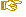i.e. the number of internal components +1, in the preprocessor we also ask the number of internal components (holes and internal edges).)
• NBRINT (I) : the number of fixed internal points
• IOPT (I) : internal point creation option:
• 0 : the mesh generator does not generate any internal points
• 1 : the mesh generator generates all the internal points
• -1 : the mesh generator generates internal points in addition to those points already given (NBRINT)
• 11 : mesh consisting of triangles - adaptive version

• if IOPT = 11
• NOMFIC (C) : name of the file that contains the initial mesh
• NIVEAU (I) : level of this mesh
• NOMFIC (C) : name of the file that contains the data structure B, this data structure B contains for each point of the initial mesh the size of the cells in its neighborhood
• NIVEAU (I) : level of this data structure
• Loop from K=1 to NCOMP, the number of contour components
• NTACO(K) (I) : the number of lines of component K of the contour. The first line of this component runs in the direction NEXTR1 to NEXTR2 if NTACO(K) > 0, and in the opposite direction if not.
• end of loop K
• Loop from L=1 to NBRINT, the number of fixed internal points (if > 0)
• NUMPIN(L) (I) : the internal point number L (taken from the list of points defined with keyword POIN);
• DIAM(L) (R) : the desired diameter for the triangles created around point L.
• end of loop L

Remarks:

• Two consecutive lines (on the same contour component) must have a common endpoint.
• A line cannot appear twice in the contour.
• The fixed internal points allow, on the one hand, to force the mesh to approach these points and, on the other hand, to specify the density of the mesh in their neighborhood (according to the value of DIAM).
• The parameter NPROPA is used when the internal points are created. They are distributed in a geometric manner if NPROPA is positive and in a arithmetic manner if it is negative.

• The position of the internal points is a function of the point positions on the given boundary. A fine mesh at the level of the boundary introduces a fine mesh of the interior. To dilute the influence of the data on the interior of the domain, the value NPROPA can be changed (in fact, its absolute value a). From a, the propagation coefficient is calculated: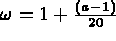.

Therefore, a=1 has a neutral effect; for a=2,3,4,5, etc., we obtain successively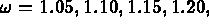etc.

• In its standard version (non adaptative) internal edges can be specified, these edges can intersect the components.

• During the use of the adaptative version the user has to give the following supplementary data
• the description of the domain : points and lines that describe the components (reference of the points must be negative)
• an initial mesh of the domain
• a data structure B that contains for each point of the initial mesh the size of the cells in its neighborhood

MANU : Calls the mesh generator CONOPO which corresponds to a manual description , i.e., from points (keyword POIN).

• MANU  (A) : the keyword to activate
• IMPRE (I) : print parameter for execution
• LEVEL (I) : the level of the resulting D.S.
• NE (I) : the number of elements to create
• Loop from J=1 to NE, the number of elements
• NCGE (I) : type of element: 1point, 2segment, 3triangle,
4quadrangle
• NUDSD (I) : the sub-domain number of the element
• Loop from K=1 to the number of element vertices
.
NUMSOM(K) (I) : the number of element vertices

• end of loop K
• Loop from L=1 to the number of element edges (if NTYP > 2)
.
NREFA(L) (I) : the reference number of the element edges

• end of loop L
• end of loop J

Remarks:

• NUMSOM(*) is one of the points created with keyword POIN.
• The order of the vertices and edges is the standard order of the D.S. NOPO.
• The edge reference numbers allows an eventual re-triangulation (keyword RETR) with projections on a curve (FFRONT or function parser).

OBJE : Calls the mesh generator CONOPO for the case of a single element.

• OBJE  (A) : the keyword to activate
• IMPRE (I) : print parameter for execution
• LEVEL (I) : the level of the resulting D.S.
• NCGE (I) : the type of element: 1point, 2segment, 3triangle,
4quadrangle
• IREF (I) : reference option
• 0 : all references are set to 0
• 1 : the references are different: for example, for a triangle:
1. for the vertices: 1, 2, 3
2. for the edges: 4, 5, 6.
whereas for a quadrangle, we have:
1. for the vertices: 1, 2, 3, 4
2. for the edges: 5, 6, 7, 8.

Remarks:

• The sub-domain number is set to 1.
• The keyword NUME can be used to modify the numbers.
• This module is used when doing simple tests quickly.

BARR : Calls the mesh generator CONOPO to create segments  from the contour data defined in terms of lines.

• BARR  (A) : the keyword to activate
• IMPRE (I) : print parameter for execution
• LEVEL (I) : level of the resulting D.S.
• NUDSD (I) : the sub-domain number to assign to the elements created
• NBRELI (I) : the number of contour lines of the domain
• NS1L (I) : the direction of the first contour line: if NS1L = 1, the line runs from endpoint NEXTR1 towards endpoint NEXTR2, if NS1L = -1, the line runs in the opposite direction (i.e. from NEXTR2 to NEXTR1)
• Loop from J=1 to NBRELI, the number of contour lines
• NOLIG(J) (I) : the number of line J
• end of loop J

Remarks:

• The contour may be closed, or not.
• The lines are consecutive.

TRAN : Calls module MODNOP to generate a mesh resulting from a translation  of a given mesh.

• TRAN  (A) : the keyword to activate
• IMPRE (I) : print parameter for execution
• LEVEL1 (I) : level of the initial D.S.
• LEVEL2 (I) : level of the resulting D.S.
• NBNNF (I) : the number of reference numbers to change
• NBNNSD (I) : the number of sub-domain numbers to change
• Loop from J=1 to NBNNF (if NBNNF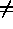0)
• NOLD(J) (I) : the reference number to change
• NNEW(J) (I) : the number to assign to items referenced by NOLD(J)
• end of loop J
• Loop from J=1 to NBNNSD (if NBNNSD0)
• NOLD(J) (I) : the sub-domain number to change
• NNEW(J) (I) : the number to assign to items referenced by NOLD(J)
• end of loop J
• TRANX (R) : the x component of the translation
• TRANY (R) : the y component of the translation

Remark:

• If a reference number indicates the presence of a curve, the coherence between the initial curve and the transformed curve is checked.

ROTA : Calls module MODNOP to generate a mesh resulting from a rotation  of a given mesh.

• ROTA  (A) : the keyword to activate
• IMPRE (I) : print parameter for execution
• LEVEL1 (I) : level of the initial D.S.
• LEVEL2 (I) : level of the resulting D.S.
• NBNNF (I) : the number of reference numbers to change
• NBNNSD (I) : the number of sub-domain numbers to change
• Loop from J=1 to NBNNF (if NBNNF0)
• NOLD(J) (I) : the reference number to change
• NNEW(J) (I) : the number to assign to items referenced by NOLD(J)
• end of loop J
• Loop from J=1 to NBNNSD (if NBNNSD0)
• NOLD(J) (I) : the sub-domain number to change
• NNEW(J) (I) : the number to assign to items referenced by NOLD(J)
• end of loop J
•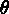(R) : the rotation angle in degrees
• XC (R) : the abscissa of the center of rotation
• YC (R) : the ordinate

Remark:

• If a reference number indicates the presence of a curve, the coherence between the initial curve and transformed curve is checked.

SYMD : Calls module MODNOP to generate the mesh resulting from symmetry  with respect to a line in a given mesh.

• SYMD  (A) : the keyword to activate
• IMPRE (I) : print parameter for execution
• LEVEL1 (I) : level of the initial D.S.
• LEVEL2 (I) : level of the resulting D.S.
• NBNNF (I) : the number of reference numbers to change
• NBNNSD (I) : the number of sub-domain numbers to change
• Loop from J=1 to NBNNF (if NBNNF0)
• NOLD(J) (I) : the reference number to change
• NNEW(J) (I) : the number to assign to items referenced by NOLD(J)
• end of loop J
• Loop from J=1 to NBNNSD (if NBNNSD0)
• NOLD(J) (I) : the sub-domain number to change
• NNEW(J) (I) : the number to assign to items referenced by NOLD(J)
• end of loop J
• A (R) : the parameters defining the line of symmetry given in the form
AX + BY + C = 0.
• B (R) : the second coefficient of the line equation
• C (R) : the third coefficient

Remark:

• If a reference number indicates the presence of a curve, the coherence between the initial curve and transformed curve is checked.

DILA : Calls module MODNOP to generate the mesh resulting from a dilation  of given mesh.

• DILA  (A) : the keyword to activate
• IMPRE (I) : print parameter for execution
• LEVEL1 (I) : level of the initial D.S.
• LEVEL2 (I) : level of the resulting D.S.
• NBNNF (I) : the number of reference numbers to change
• NBNNSD (I) : the number of sub-domain numbers to change
• Loop from J=1 to NBNNF (if NBNNF0)
• NOLD(J) (I) : the reference number to change
• NNEW(J) (I) : the number to assign to items referenced by NOLD(J)
• end of loop J
• Loop from J=1 to NBNNSD (if NBNNSD0)
• NOLD(J) (I) : the sub-domain number to change
• NNEW(J) (I) : the number to assign to items referenced by NOLD(J)
• end of loop J
• DILX (R) : the dilation coefficient in the x direction
• DILY (R) : the dilation coefficient in the y direction
• XINV (R) : the abscissa of a point which is invariant by this transformation
• YINV (R) : the ordinate

Remark:

• If a reference number indicates the presence of a curve, the coherence between the initial curve and transformed curve is checked.

Q4T  : Calls module QUATRI to split  each quadrangle of a given mesh into four triangles.

• Q4T (A) : the keyword to activate
• IMPRE (I) : print parameter for execution
• LEVEL1 (I) : level of the initial D.S.
• LEVEL2 (I) : level of the resulting D.S.

RETR : Calls module RETRIN to split  each element of a given mesh into sub-elements of the same type.

• RETR  (A) : the keyword to activate
• IMPRE (I) : print parameter for execution
• LEVEL1 (I) : level of the initial D.S.
• LEVEL2 (I) : level of the resulting D.S.
• N (I) : the splitting parameter

Remarks:

• Each element is split into N� elements of the same type (or N in the case of a segment).
• This module is capable of projecting the points, which it generates when encountering a reference (or edge) number associated with a curve, onto the curved boundary.
• For N=-1 and a mesh consisting of triangles only, we create the barycentre and each triangle is split into three sub-triangles using this point.

REGU : Calls module REGMA2 to regularize  a given mesh (by barycentering).

• REGU  (A) : the keyword to activate
• IMPRE (I) : print parameter for execution
• LEVEL1 (I) : level of the initial D.S.
• LEVEL2 (I) : level of the resulting D.S.

For IMPRE5, a histogram of the triangular element qualities are given, before and after regularization (the quality of a triangle is defined by the value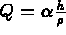where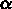is a normalization coefficient such that Q=1 for an equilateral triangle, h is the diameter of the measured element, and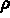is the radius of its inscribed circle. We note that Q increases as the triangle flattens.

AIGU : Calls module AIGUNO to remove the obtuse  angles in a mesh composed of triangles.

• AIGU  (A) : the keyword to activate
• IMPRE (I) : print parameter for execution
• LEVEL1 (I) : level of the initial D.S.
• LEVEL2 (I) : level of the resulting D.S.

AFFL : Calls module AFFNOP to refine  a given mesh locally, around certain vertices.

• AFFL  (A) : the keyword to activate
• IMPRE (I) : print parameter for execution
• LEVEL1 (I) : level of the initial D.S.
• LEVEL2 (I) : level of the resulting D.S.
1 : generate triangles when splitting the quadrangles
0 : if not
• LSAT (I) : the number of vertices to process
• Loop from J=1 to LSAT
• NUMSOM(J) (I) : the number of vertex J around which to refine
• end of loop J

Remark:

• Each vertex is counted and specified as many times as we desire to refine its neighborhood.

NUME : Calls module MODNOP to modify attributes (reference or sub-domain numbers) in a given mesh.

• NUME  (A) : the keyword to activate
• IMPRE (I) : print parameter for execution
• LEVEL1 (I) : level of the initial D.S.
• LEVEL2 (I) : level of the resulting D.S.
• NBNNF (I) : the number of reference numbers to change
• NBNNSD (I) : the number of sub-domain numbers to change
• Loop from J=1 to NBNNF (if NBNNF0)
• NOLD(J) (I) : the reference number to change
• NNEW(J) (I) : the number to assign to items referenced by NOLD(J)
• end of loop J
• Loop from J=1 to NBNNSD (if NBNNSD0)
• NOLD(J) (I) : the sub-domain number to change
• NNEW(J) (I) : the number to assign to items referenced by NOLD(J)
• end of loop J

Remark:

• If a reference number indicates the presence of a curve, the coherence between the initial curve and transformed curve is checked (at the reference number level).

RECO : Calls module RECOLC to "glue together"  two meshes to form a new mesh.

• RECO  (A) : the keyword to activate
• IMPRE (I) : print parameter for execution
• LEVEL1 (I) : level of the first initial D.S.
• LEVEL2 (I) : level of the second initial D.S.
• LEVEL3 (I) : level of the resulting D.S.
• EPS (R) : the precision of the union (for example 0.01)
• IOPT (I) : the gluing option: 0 geometrical, 1 physical.
• NBNNF (I) : the number of reference numbers to change
• NBNNSD (I) : the number of sub-domain numbers to change
• Loop from J=1 to NBNNF (if NBNNF0)
• NOLD(J) (I) : the reference numbers to change
• NNEW(J) (I) : the number to assign to items referenced by NOLD(J)
• end of loop J
• Loop from J=1 to NBNNSD (if NBNNSD0)
• NOLD(J) (I) : the sub-domain number to change
• NNEW(J) (I) : the number to assign to items referenced by NOLD(J)
• end of loop J

Remarks:

• A geometrical "gluing together" consists of identifying the points by taking only their positions into account.
• On the contrary, a physical "gluing together" consists of identifying the points by taking their positions and reference numbers into account (two points are said identical if their positions and references are the same).

"Cracks" can be generated by selecting an appropriate gluing option.

ADPO : Calls module ADPNOP to define the nodes  of a given mesh (as opposed to a mesh for which the nodes are identical to the vertices).

• ADPO  (A) : the keyword to activate
• IMPRE (I) : print parameter for execution
• LEVEL1 (I) : level of the initial D.S.
• LEVEL2 (I) : level of the resulting D.S.
• N1 (I) : the number of nodes on the sides (endpoints included)
• ISET (I) : the number of internal nodes in each triangle
• ISEQ (I) : the number of internal nodes in each quadrangle
• NOESOM (I) :
1 : the vertices are the nodes
0 : if not

Remarks:

• It is no longer possible to activate the preceding keywords, consequently this keyword is called once the entire geometry has been processed.
• Renumbering is desired after this operation.

EXTR : Calls module TRCOAC to construct a D.S. NOPO containing edges with a given reference number.

• EXTR  (A) : the keyword to activate
• IMPRE (I) : print parameter for execution
• LEVEL1 (I) : level of the initial D.S.
• LEVEL2 (I) : level of the resulting D.S.
• NBNNF (I) : the number of edge reference numbers to consider
• Loop from J=1 to NBNNF (if NBNNF0)
• NREF(J) (I) : the edge reference numbers to retain
• end of loop J

RENC : Calls module GIBBS to renumber  the nodes of a mesh.

• RENC  (A) : the keyword to activate
• IMPRE (I) : print parameter for execution
• LEVEL1 (I) : level of the initial D.S.
• LEVEL2 (I) : level of the resulting D.S.

Remark:

• This keyword usually comes just before saving the final mesh on file.

RENE : Calls module GIBBS to renumber  the elements and the nodes of a mesh.

• RENE  (A) : the keyword to activate
• IMPRE (I) : print parameter for execution
• LEVEL1 (I) : level of the initial D.S.
• LEVEL2 (I) : level of the resulting D.S.

Remark:

• This keyword usually comes just before saving the final mesh on file.

COUL : Calls module COULE1 to renumber  the elements by coloring.

• COUL  (A) : the keyword to activate
• IMPRE (I) : print parameter for execution
• LEVEL1 (I) : level of the initial D.S.
• LEVEL2 (I) : level of the resulting D.S.
• LVECT (I) : the maximum number of elements per color
• NLC (I) : the number of curved boundaries described
• NOMTAB (A) : the name of the array to be associated with the D.S.
• Loop from J=1 to NLC, the number of curved lines
• NREF(J) (I) the reference number of line J
• end of loop J

Remark:

• This keyword is used to regroup the elements of a mesh into disjoint sets. Consequently, it enables us to perform a calculation, for example of element matrices, on super-calculators.

SAUV : Calls module SAUVER to store  a mesh, residing in main memory, on file.

• SAUV  (A) : the keyword to activate
• IMPRE (I) : print parameter for execution
• NINOPO (I) : level of the D.S. to be saved on file
• NTNOPO (I) : the number of arrays to associate to the D.S. (usually 0)
• NOM (A) : the file name
• Loop from J=1 to NTNOPO (if NTNOPO0)
• NOMTAB (A) : the name of array J (4 characters)
• NTYP (I) : its type
• LONG (I) : the number of words
• Loop from K=1 to the number of variables
• VAL(K) (NTYP) : the variables of the array
• end of loop K
• COMMENT (A) : comment regarding the contents of array J
• end of loop J

Remarks:

• This keyword may be activated at any time to save a partial or final mesh.
• The possible curves defined by the function parser are stored automatically in the D.S. by means of associated arrays.

INTR : Calls module SDREST to introduce  a mesh residing on file into main memory.

• INTR  (A) : the keyword to activate
• IMPRE (I) : print parameter for execution
• NINOPO (I) : the level of the D.S. to introduce into m.m.
• NOM (A) : the name of the file containing the D.S.

Remarks:

• In order to be usable by the geometrical transformation modules, a mesh introduced in this way must be of type P1, i.e., its nodes and vertices must coincide.
• The possible curves defined by the function parser are restored automatically and added to those already present.

TUER : Calls module TUERSD to remove one or several mesh data structures (D.S.) from memory.

• TUER  (A) : the keyword to activate
• IMPRE (I) : print parameter for execution
• NBRE (I) : the number of D.S. to "kill"
• Loop from J=1 to NBRE
• LEVEL(J) (I) : the level of D.S. J to delete from the arrays
• end of loop J

Remark:

• This keyword can be activated to economize on memory space.

IMPR : Call module IMNOPO to print  the contents of a mesh data structure (D.S.).

• IMPR  (A) : the keyword to activate
• IMPRE (I) : print parameter for execution
• NINOPO (I) : the level of the D.S. to print

DESS : Calls module TRNOPO to plot  a mesh.

• DESS  (A) : the keyword to activate
• ITERM1 (I) : the terminal number in the nomenclature of your installation of the software package F3D
• ITERM2 (I) : set 0
• IOPT (I) : the mesh plotting option: 1 the mesh, 2 the contour, 3 the referenced boundaries, 4 the shrunken mesh.
• LEVEL (I) : the level of the D.S. to plot

Remarks:

• The couple (ITERM1, ITERM2) defines the graphics device.
• The preprocessor TRNOXX is more frequently used to plot meshes.
• Consequently, by deleting this keyword from the APNOPO facilities, we avoid loading the graphics libraries at the time the meshes are conceived.

FIN : Request to terminate the job.

• FIN  (A) : the keyword to activate

In addition, the following keywords are found in preprocessor APNOXX:

LIRE
: request to read  another data file (of the same format).
CONT
: request to suppress the data coherence controls .